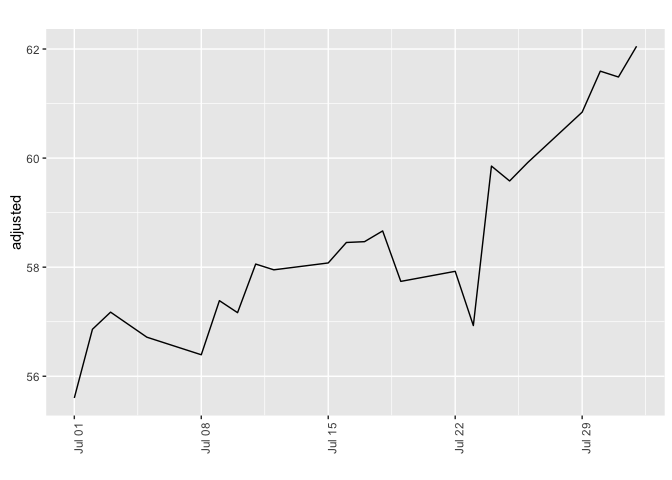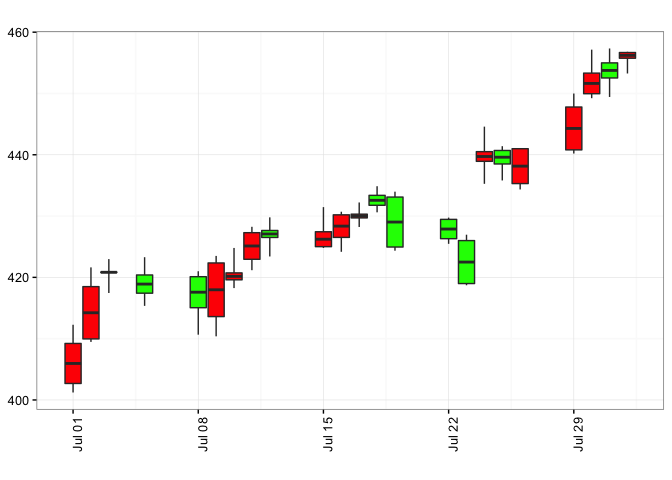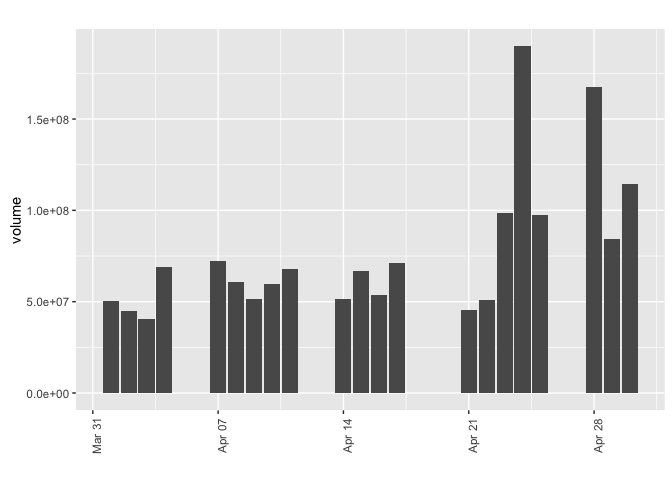# Examples of using FinCal

| category Finance  | tag R  Finance

Updated on Tue Dec 29 10:47:56 2015

## FinCal – Time Value of Money, time series analysis and Computational Finance

FinCal is available on [CRAN] (http://cran.r-project.org/web/packages/FinCal/) and GitHub

install.packages("FinCal",dependencies=TRUE) # from CRAN


or

library("devtools")
install_github("felixfan/FinCal") # from GitHub

library(FinCal)

Note: for all examples, cash inflows are positive and outflows are negative.


Examples for version 0.1 -0.6

## Example 1 Computing effective annual rate (EAR)

Using a stated rate of 4.25%, compute EARs for semiannual, quarterly, monthly, daily and continuous compounding.

ear(0.0425,2)

 0.04295156

ear(0.0425,4)

 0.04318215

ear(0.0425,12)

 0.04333772

ear(0.0425,365)

 0.04341347

ear.continuous(0.0425)

 0.04341606


Calculate the FV of a $500 investment at the end of ten years if it earns an annually compounded rate of return of 6%. fv.simple(r=0.06,n=10,pv=-500)   895.4238  ## Example 3 PV of a single sum Given a discount rate of 3%, calculate the PV of a$1,000,000 cash flow that will be received in five years.

pv.simple(r=0.03,n=5,fv=1000000)

 -862608.8


## Example 4 FV of an ordinary annuity and annuity due

What is the future value of an ordinary annuity that pays $15,000 per year at the end of each of the next 25 years, given the investment is expected to earn a 6% rate of return? fv.annuity(r=0.06,n=25,pmt=-15000,type=0)   822967.7  What is the future value of an annuity that pays$10,000 per year at the beginning of each of the next three years, commencing today, if the cash flows can be invested at an annual rate of 5%?

fv.annuity(r=0.05,n=3,pmt=-10000,type=1)

 33101.25


## Example 5 PV of an ordinary annuity and annuity due

What is the PV of an annuity that pays $20,000 per year at the end of each of the next 25 years, given a 6% discount rate? pv.annuity(r=0.06,n=25,pmt=-20000,type=0)   255667.1  Given a discount rate of 10%, what is the present value of a 10-year annuity that makes a series of$1000 payments at the beginning of each of the next three years, starting today?

pv.annuity(r=0.1,n=10,pmt=-1000,type=1)

 6759.024


A preferred stock that will pay $2.50 per year in annual dividends beginning next year and plans to follow this dividend policy forever. Given an 10% rate of return, what is the value of this preferred stock today? pv.perpetuity(r=0.1,pmt=2.5,type=0)   -25  ## Example 7 Rate of return for a perpetuity Using the preferred stock described in the preceding example, determine the rate of return that an investor would realize if she paid$75 per share for the stock.

r.perpetuity(pmt=2.5,pv=-75)

 0.03333333


## Example 8 PV of a bond’s cash flows

A bond will make coupon interest payments of 70 HK$(7% of its face value) at the end of each year and will also pay its face value of 1,000 HK$ at maturity in 10 years. If the appropriate discount rate is 6%, what is the present value of the bond’s promised cash flows?

pv(r=0.06,n=10,fv=1000,pmt=70,type=0)

 -1073.601


## Example 9 Computing the FV and PV of an uneven cash How series

Using a rate of return of 6%, compute the future value of the 6-year uneven cash flow stream occured at the end of each year. (-10000 -5000 2000 4000 6000 8000)

fv.uneven(r=0.06, cf=c(-10000, -5000, 2000, 4000, 6000, 8000))

 -1541.791


Compute the present value of this 6-year uneven cash How stream described above using a 10% rate of return.

pv.uneven(r=0.1, cf=c(-10000, -5000, 2000, 4000, 6000, 8000))

 747.1377


A company plans to borrow $500,000 for five years. The company’s bank will lend the money at a rate of 6% and requires that the loan be paid off in five equal end-of-year payments. Calculate the amount of the payment that the company must make in order to fully amortize this loan in five years. pmt(r=0.06,n=5,pv=500000,fv=0)   -118698.2  ## Example 11 Computing the number of periods in an annuity How many$1000 end-of-year payments are required to accumulate $10,000 if the discount rate is 9%? n.period(r=0.09,pv=0,fv=10000,pmt=-1000,type=0)   7.448028  ## Example 12 Computing the rate of return for a period Suppose you have the opponunity to invest$1000 at the end of each of the next five years in exchange for $6000 at the end of the fifth year. What is the annual rate of return on this investment? discount.rate(n=5,fv=6000,pmt=-1000,pv=0,type=0)   0.09130091  ## Example 13 Computing NPV Calculate the NPV of an investment project with an initial cost of$6 million and positive cash flows of $2.6 million at the end of Year 1,$2.4 million at the end of Year 2, and $3.8 million at the end ofYear 3. Use 8% as the discount rate. npv(r=0.08, cf=c(-6,2.6,2.4,3.8))   1.481583  ## Example 14 Computing IRR What is the IRR for the investment described in example 13? irr(cf=c(-6,2.6,2.4,3.8))   0.2032579  ## Example 15 Computing HPR Suppose a stock is purchased for$3 and is sold for $4 six months later, during which time it paid$0.50 in dividends. What is the holding period return?

hpr(ev=4,bv=3,cfr=0.5)

 0.5


## Example 16 Computing time-weighted rate of return

An investor purchases a share of stock at t = 0 for $10. At the end of the year, t = 1 , the investor buys another share of the same stock for$12. At the end of Year 2, the investor sells both shares for $13 each. At the end of both years 1 and 2, the stock paid a$1 per share dividend. What is the annual time-weighted rate of return for this investment?

twrr(ev=c(12,26),bv=c(10,24),cfr=c(1,2))

 0.2315302


## Example 17 Computing Bank discount yield

Calculate the bank discount yield for a T-hill priced at $9,850, with a face value of$10,000 and 120 days until maturity.

bdy(d=150,f=10000,t=120)

 0.045


## Example 18 Convert holding period return to the effective annual rate

Compute the EAY using the 120-day HPY of 2.85%.

hpr2ear(hpr=0.0285,t=120)

 0.08923453


## Example 19 Computing money market yield

What is the money market yield for a 120-day T-bill that has a bank discount yield of 4.50%?

bdy2mmy(bdy=0.045,t=120)

 0.04568528


## Example 20 Converting among EAR, HPY, and MMY

Assume the price of a $10,000 T-hill that matures in 150 days is$9,800. The quoted money market yield is 4.898%. Compute the HPY and the EAR.

hpr(ev=10000,bv=9800)

 0.02040816

mmy2hpr(mmy=0.04898,t=150)

 0.02040833

hpr2ear(hpr=mmy2hpr(mmy=0.04898,t=150),t=150)

 0.05038874

ear2hpr(ear=hpr2ear(hpr=mmy2hpr(mmy=0.04898,t=150),t=150),t=150)

 0.02040833


## Example 21 Bond-equivalent yield calculation

What is the yield on a bond-equivalent basis of a 3-month loan has a holding period yield of 4%?

hpr2bey(hpr=0.04,t=3)

 0.1632


What is the yield on a bond-equivalent basis of an investment with 6% effective annual yield?

ear2bey(ear=0.06)

 0.05912603


## Example 22 Weighted mean as a portfolio return

A portfolio consists of 40% common stocks, 50% bonds, and 10% cash. If the return on common stocks is 9%, the return on bonds is 6%, and the return on cash is 1%, what is the portfolio return?

wpr(r=c(0.09, 0.06, 0.01),w=c(0.4,0.5,0.1))

 0.067


or

rs=c(0.09, 0.06, 0.01)
ws=c(0.4,0.5,0.1)
wpr(r=rs,w=ws)

 0.067


## Example 23 Geometric mean return

For the last three years, the returns for Acme Corporation common stock have been -5%, 11%, and 9%. Compute the compound annual rate of return over the 3-year period.

geometric.mean(r=c(-0.05, 0.11, 0.09))

 0.04750883


## Example 24 Calculating average cost with the harmonic mean

An investor purchases $10,000 of stock each month, and over the last three months the prices paid per share were$4.5, $5.2, and$4.8. What is the average cost per share for the shares acquired?

harmonic.mean(p=c(4.5,5.2,4.8))

 4.816467


apple <- get.ohlc.yahoo(symbol="AAPL",start="2013-07-01",end="2013-08-01")

date   open   high    low  close    volume adjusted
23 2013-07-01 402.69 412.27 401.22 409.22  97763400 55.60200
22 2013-07-02 409.96 421.63 409.47 418.49 117466300 56.86155
21 2013-07-03 420.86 422.98 417.45 420.80  60232200 57.17542
20 2013-07-05 420.39 423.29 415.35 417.42  68506200 56.71616
19 2013-07-08 420.11 421.00 410.65 415.05  74534600 56.39415
18 2013-07-09 413.60 423.50 410.38 422.35  88146100 57.38602


apple <- get.ohlc.google(symbol="AAPL",start="2013-07-01",end="2013-08-01")

date  open  high   low close    volume
23 2013-07-01 57.53 58.90 57.32 58.46  97793045
22 2013-07-02 58.57 60.23 58.50 59.78 117521579
21 2013-07-03 60.12 60.43 59.64 60.11  60232158
20 2013-07-05 60.06 60.47 59.34 59.63  68520760
19 2013-07-08 60.02 60.14 58.66 59.29  74578420
18 2013-07-09 59.09 60.50 58.63 60.34  88172238


applespy <- get.ohlcs.yahoo(symbols=c("AAPL","SPY"),start="2013-01-01",end="2013-07-31")
head(applespy$AAPL)  date open high low close volume adjusted 146 2013-01-02 553.82 555.00 541.63 549.03 140129500 73.67851 145 2013-01-03 547.88 549.67 541.00 542.10 88241300 72.74852 144 2013-01-04 536.97 538.63 525.83 527.00 148583400 70.72214 143 2013-01-07 522.00 529.30 515.20 523.90 121039100 70.30613 142 2013-01-08 529.21 531.89 521.25 525.31 114676800 70.49535 141 2013-01-09 522.50 525.01 515.99 517.10 101901100 69.39359  head(applespy$SPY)

date   open   high    low  close    volume adjusted
146 2013-01-02 145.11 146.15 144.73 146.06 192059000 137.5859
145 2013-01-03 145.99 146.37 145.34 145.73 144761800 137.2750
144 2013-01-04 145.97 146.61 145.67 146.37 116817700 137.8779
143 2013-01-07 145.85 146.11 145.43 145.97 110002500 137.5011
142 2013-01-08 145.71 145.91 144.98 145.55 121265100 137.1055
141 2013-01-09 145.87 146.32 145.64 145.92  90745600 137.4540


all <- get.ohlcs.google(symbols=c("YHOO","SPY", "SINA"),start="2013-01-01",end="2013-07-31")
head(all$YHOO)  date open high low close volume 146 2013-01-02 20.20 20.32 20.01 20.08 20463033 145 2013-01-03 20.05 20.10 19.72 19.78 19599094 144 2013-01-04 19.76 19.95 19.72 19.86 12489700 143 2013-01-07 19.56 19.58 19.28 19.40 23866609 142 2013-01-08 19.32 19.68 19.30 19.66 16932176 141 2013-01-09 19.73 19.75 19.22 19.33 21656278  head(all$SPY)

date   open   high    low  close    volume
146 2013-01-02 145.11 146.15 144.73 146.06 192058911
145 2013-01-03 145.99 146.37 145.34 145.73 144761781
144 2013-01-04 145.97 146.61 145.67 146.37 116817675
143 2013-01-07 145.85 146.44 145.43 145.97 110002444
142 2013-01-08 145.71 145.91 144.98 145.55 121265078
141 2013-01-09 145.87 146.32 145.64 145.92  90745581

head(all$SINA)  date open high low close volume 146 2013-01-02 52.24 55.19 51.75 52.27 3947513 145 2013-01-03 52.34 53.61 51.54 52.77 2200712 144 2013-01-04 52.70 52.94 51.70 52.76 1466652 143 2013-01-07 51.81 52.67 51.40 52.57 1368991 142 2013-01-08 52.20 53.00 51.41 51.81 1381026 141 2013-01-09 51.76 52.43 50.61 51.54 2098518  ## Example 26 Plots open-high-low-close chart of (financial) time series. Line chart apple <- get.ohlc.yahoo(symbol="AAPL",start="2013-07-01",end="2013-08-01") lineChart(apple,y="adjusted")Candlestick chart apple <- get.ohlc.yahoo(symbol="AAPL",start="2013-07-01",end="2013-08-01") candlestickChart(apple,y="adjusted")Volume chart apple <- get.ohlc.yahoo("AAPL",start="2014-04-01",end="2014-04-30") volumeChart(apple,y="adjusted")Multiple line chart all <- get.ohlcs.yahoo(c("SBUX","AAPL","SPY"),start="2013-01-01",end="2013-06-30") lineChartMult(all,y="adjusted")## Examples 27 Inventory Expense Recognition – cost of goods sold Three methods: first-in, first-out (FIFO) method; last-in, first-out (LIFO) method; weighted average cost method. e.g. during one month beginning inventory: 20 units @$2/unit buy : 30 units @ $3/unit buy : 50 units @$5/unit sold : 70 units what is the cost of goods sold using three menthod?

# first-in, first-out (FIFO) method
cogs(uinv=20,pinv=2,units=c(30,50),price=c(3,5),sinv=70,method="FIFO")

$costOfGoods  230$endingInventory
 150

# last-in, first-out (LIFO) method
cogs(uinv=20,pinv=2,units=c(30,50),price=c(3,5),sinv=70,method="LIFO")

$costOfGoods  310$endingInventory
 70

# weighted average cost method
cogs(uinv=20,pinv=2,units=c(30,50),price=c(3,5),sinv=70,method="WAC")

$costOfGoods  266$endingInventory
 114


## Example 28 Depreciation Expense Recognition

Methods: straight line depreciation method, double-declining balance (DDB) e.g., One Company recently purchased a machine at a cost of $9,800. The machine is expected to have a residual value of$2,000 at the end of its useful life in five years. What is the depreciation expense for all five years using two different menthods?

# straight line depreciation method
slde(cost=9800,rv=2000,t=5)

 1560

# double-declining balance (DDB)
ddb(cost=9800,rv=2000,t=5)

t    ddb
[1,] 1 3920.0
[2,] 2 2352.0
[3,] 3 1411.2
[4,] 4  116.8
[5,] 5    0.0


## Example 29 Weighted average shares and EPS

One company has net income of $100,000 and paid$10,000 cash dividends to its preferred shareholders and $10,750 cash dividends to its common shareholders. At the beginning of the year, there were 20,000 shares of common stock outstanding. 20,000 new shares were issued on July 1 . what is the weighted average shares and basic EPS? # weighted average shares was=was(ns=c(20000,20000),nm=c(12,6)) was   30000  # basic EPS EPS(ni=100000,pd=10000,w=was)   3  One company has 15,000 shares outstanding all year. It had 2,000 outstanding warrants all year, convertible into one share each at$20 per share. The year-end price of stock was $40, and the average stock price was$30. What effect will these warrants have on the weighted average number of shares?

iss(amp=30,ep=20,n=2000)

 666.6667


During 2013, X reported net income of $231,200 and had 400,000 shares of common stock outstanding for the entire year. X had 2,000 shares of 10%,$100 par convertible preferred stock, convertible into 40 shares each, outstanding for the entire year. X also had 1200, 7%, $1,000 par value convertible bonds, convertible into 100 shares each, outstanding for the entire year. Finally, X had 20,000 stock options outstanding during the year. Each option is convertible into one share of stock at$15 per share. The average market price of the stock for the year was \$20. The tax rate is 40%. What are X’s basic and diluted EPS?

EPS(ni=231200,pd=2000*0.1*100,w=400000)

 0.528

iss=iss(amp=20,ep=15,n=20000)
diluted.EPS(ni=231200,pd=2000*0.1*100,cpd=2000*0.1*100,cdi=1200*0.07*1000,tax=0.4,w=400000,cps=2000*40,cds=1200*100,iss=iss)

 0.4654545